# 1 Transformations of the Euclidean space

In the three-dimensional extended Euclidean space ${}_{\infty }{\mathbf{E}}^{3}\left(R\right)$ with the defined homogeneous coordinate system, a group of Euclidean metric transformations can be determined on the subset of all real points ${\mathbf{E}}^{3}\left(R\right)$. Any real point $A=\phantom{\rule{mediummathspace}{0.2em}}$$\left(x,y,z,1\right)$ in the subspace can be attached its image $A\text{'}=\left(x\text{'},y\text{'},z\text{'},1\right)$, while the relation of coordinates can be expressed in the following way

 $A\text{'}=A.{\mathrm{T}}_{\mathrm{M}}$

where ${\mathrm{T}}_{\mathrm{M}}\phantom{\rule{mediummathspace}{0.2em}}$ is the regular square matrix of rank 4.

We recognize these metric transformations of the space: identity, symmetry about a point - central symmetry, symmetry about a line - axial symmetry, symmetry about a plane - plane reflection, revolution about a line, translation, translated symmetry about a line, translated symmetry about a line or a plane, revolved symmetry about a plane, helical movement.

## 1.1 Identity

Equations and matrix of identity

 $x\text{'}=x$
 $y\text{'}=y$
 $z\text{'}=z$
 $\mathrm{I}=\left(\begin{array}{cccc}1& 0& 0& 0\\ 0& 1& 0& 0\\ 0& 0& 1& 0\\ 0& 0& 0& 1\end{array}\right)$

## 1.2 Symmetry about a point

Let the centre of symmetry be in the origin O, then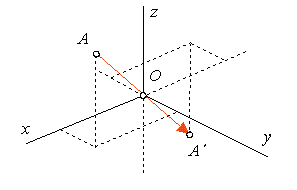Figure 1: Symmetry about origin

Equation and matrix of central symmetry about O

 $x\text{'}=-x$
 $y\text{'}=-y$
 $z\text{'}=-z$
 ${\mathrm{T}}_{\mathrm{M}}=\left(\begin{array}{cccc}-1& 0& 0& 0\\ 0& -1& 0& 0\\ 0& 0& -1& 0\\ 0& 0& 0& 1\end{array}\right)$

## 1.3 Symmetry about a line

Let the axis of symmetry be in the coordinate axis x, then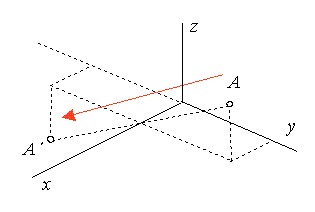Figure 2: Symmetry about axis x

Equations and matrix of the axial symmetry about x

 $x\text{'}=x$
 $y\text{'}=-y$
 $z\text{'}=-z$
 ${\mathrm{T}}_{\mathrm{M}}=\left(\begin{array}{cccc}1& 0& 0& 0\\ 0& -1& 0& 0\\ 0& 0& -1& 0\\ 0& 0& 0& 1\end{array}\right)$

Let the axis of symmetry be in the coordinate axis y, then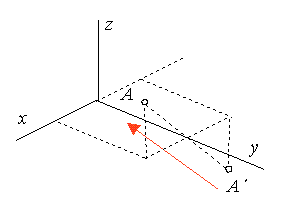Figure 3: Symmetry about axis y

Equations and matrix of the axial symmetry about y

 $x\text{'}=-x$
 $y\text{'}=y$
 $z\text{'}=-z$
 ${\mathrm{T}}_{\mathrm{M}}=\left(\begin{array}{cccc}-1& 0& 0& 0\\ 0& 1& 0& 0\\ 0& 0& -1& 0\\ 0& 0& 0& 1\end{array}\right)$

Let the axis of symmetry be in the coordinate axis z, then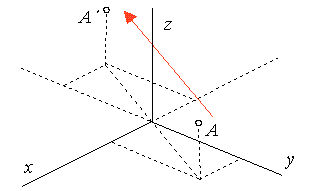Figure 4: Symmetry about axis z

Equations and matrix of the axial symmetry about z

 $x\text{'}=-x$
 $y\text{'}=-y$
 $z\text{'}=z$
 ${\mathrm{T}}_{\mathrm{M}}=\left(\begin{array}{cccc}-1& 0& 0& 0\\ 0& -1& 0& 0\\ 0& 0& 1& 0\\ 0& 0& 0& 1\end{array}\right)$

## 1.4 Symmetry about a plane

Let the plane of symmetry be in the coordinate plane $\pi =xy$, then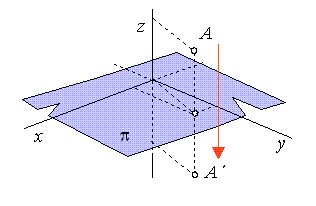Figure 5: Symmetry about plane xy

Equations and matrix of the symmetry about plane $\pi =$xy

 $x\text{'}=x$
 $y\text{'}=y$
 $z\text{'}=-z$
 ${\mathrm{T}}_{\mathrm{M}}=\left(\begin{array}{cccc}1& 0& 0& 0\\ 0& 1& 0& 0\\ 0& 0& -1& 0\\ 0& 0& 0& 1\end{array}\right)$

Let the plane of symmetry be in the coordinate plane $v=\mathrm{xz}$, then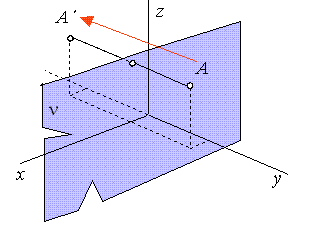Figure 6: Symmetry about plane xz

Equations and matrix of the symmetry about plane $v=\mathrm{xz}$

 $x\text{'}=x$
 $y\text{'}=-y$
 $z\text{'}=z$
 ${\mathrm{T}}_{\mathrm{M}}=\left(\begin{array}{cccc}1& 0& 0& 0\\ 0& -1& 0& 0\\ 0& 0& 1& 0\\ 0& 0& 0& 1\end{array}\right)$

Let the plane of symmetry be in the coordinate plane $\mu =\mathrm{yz}$, then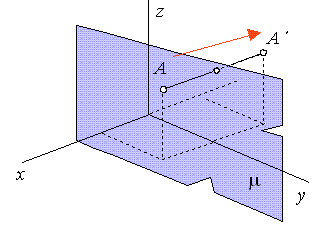Figure 7: Symmetry about plane yz

Equations and matrix of the symmetry about plane $\mu =\mathrm{yz}$

 $x\text{'}=-x$
 $y\text{'}=y$
 $z\text{'}=z$
 ${\mathrm{T}}_{\mathrm{M}}=\left(\begin{array}{cccc}-1& 0& 0& 0\\ 0& 1& 0& 0\\ 0& 0& 1& 0\\ 0& 0& 0& 1\end{array}\right)$

## 1.5 Revolution about a line

Let the axis of revolution be in the coordinate axis $z$, and angle of revolution be $\varphi$, then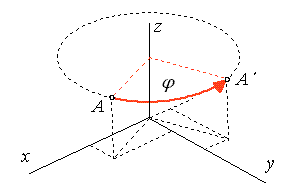Figure 8: Revolution about axis z

Equations and matrix of the revolution about axis z

 $x\text{'}=x\phantom{\rule{2mm}{2mm}}\mathrm{cos}\phantom{\rule{2mm}{2mm}}\varphi -y\phantom{\rule{2mm}{2mm}}\mathrm{sin}\phantom{\rule{2mm}{2mm}}\varphi$
 $y\text{'}=x\phantom{\rule{2mm}{2mm}}\mathrm{sin}\phantom{\rule{2mm}{2mm}}\varphi +y\phantom{\rule{2mm}{2mm}}\mathrm{cos}\phantom{\rule{2mm}{2mm}}\varphi$
 $z\text{'}=z$
 ${\mathrm{T}}_{\mathrm{M}}=\left(\begin{array}{cccc}\mathrm{cos}\phantom{\rule{2mm}{2mm}}\varphi & \mathrm{sin}\phantom{\rule{2mm}{2mm}}\varphi & 0& 0\\ -\mathrm{sin}\phantom{\rule{2mm}{2mm}}\varphi & \mathrm{cos}\phantom{\rule{2mm}{2mm}}\varphi & 0& 0\\ 0& 0& 1& 0\\ 0& 0& 0& 1\end{array}\right)$

Let the axis of revolution be in the coordinate axis $y$, and angle of revolution be $\varphi$, then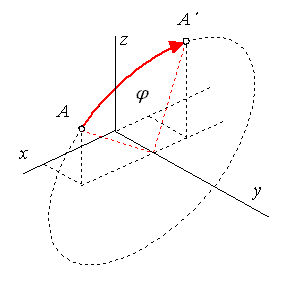Figure 9: Revolution about axis y

Equations and matrix of the revolution about axis y

 $x\text{'}=x\phantom{\rule{2mm}{2mm}}\mathrm{cos}\phantom{\rule{2mm}{2mm}}\varphi +z\phantom{\rule{2mm}{2mm}}\mathrm{sin}\phantom{\rule{2mm}{2mm}}\varphi$
 $y\text{'}=y$
 $z\text{'}=-x\phantom{\rule{2mm}{2mm}}\mathrm{sin}\phantom{\rule{2mm}{2mm}}\varphi +z\phantom{\rule{2mm}{2mm}}\mathrm{cos}\phantom{\rule{2mm}{2mm}}\varphi$
 ${\mathrm{T}}_{\mathrm{M}}=\left(\begin{array}{cccc}\mathrm{cos}\phantom{\rule{2mm}{2mm}}\varphi & 0& -\mathrm{sin}\phantom{\rule{2mm}{2mm}}\varphi & 0\\ 0& 1& 0& 0\\ \mathrm{sin}\phantom{\rule{2mm}{2mm}}\varphi & 0& \mathrm{cos}\phantom{\rule{2mm}{2mm}}\varphi & 0\\ 0& 0& 0& 1\end{array}\right)$

Let the axis of revolution be in the coordinate axis $x$, and angle of revolution be $\varphi$, then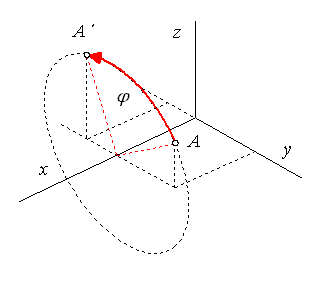Figure 10: Revolution about axis x

Equations and matrix of the revolution about axis x

 $x\text{'}=x$
 $y\text{'}=y\phantom{\rule{2mm}{2mm}}\mathrm{cos}\phantom{\rule{2mm}{2mm}}\varphi \phantom{\rule{2mm}{2mm}}-z\phantom{\rule{2mm}{2mm}}\mathrm{sin}\phantom{\rule{2mm}{2mm}}\varphi$
 $z\text{'}=y\phantom{\rule{2mm}{2mm}}\mathrm{sin}\phantom{\rule{2mm}{2mm}}\varphi +z\phantom{\rule{2mm}{2mm}}\mathrm{cos}\phantom{\rule{2mm}{2mm}}\varphi$
 ${\mathrm{T}}_{\mathrm{M}}=\left(\begin{array}{cccc}1& 0& 0& 0\\ 0& \mathrm{cos}\phantom{\rule{2mm}{2mm}}\varphi & \mathrm{sin}\phantom{\rule{2mm}{2mm}}\varphi & 0\\ 0& -\mathrm{sin}\phantom{\rule{2mm}{2mm}}\varphi & \mathrm{cos}\phantom{\rule{2mm}{2mm}}\varphi & 0\\ 0& 0& 0& 1\end{array}\right)$

## 1.6 Translation

Let the translation be given by vector $\mathbf{a}=\left(m,n,l,0\right)$, then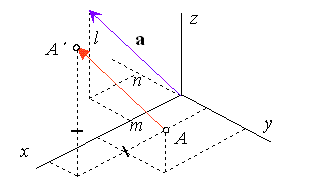Figure 11: Translation

Equations and matrix of the translation

 $x\text{'}=x+m$
 $y\text{'}=y+n$
 $z\text{'}=z+l$
 ${\mathrm{T}}_{\mathrm{M}}=\left(\begin{array}{cccc}1& 0& 0& 0\\ 0& 1& 0& 0\\ 0& 0& 1& 0\\ m& n& l& 1\end{array}\right)$